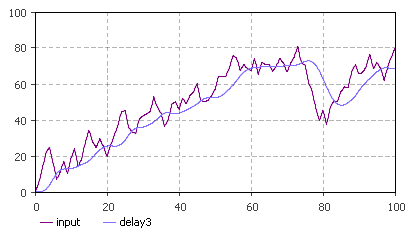AnyLogic

# delay3

delay3 is the system dynamics function that returns a third order exponential delay of the input. If the delay time changes, the input is preserved.

The plot below illustrates how the function works (initialValue = 0.0; delayTime = 5.0):This function can be called in formulas of system dynamics variables and has two notations:

• delay3(input, delayTime, initialValue)

input can be a flow variable, or a numeric expression of any complexity.

delayTime can be either a constant or a numeric expression (e.g. a function call, or a numeric parameter). It should return a positive number: delay3 function with zero or negative delay time returns NaN (not a number).

• delay3(input, delayTime)

A simplified notation of the function. It is used when the initialValue is zero.

#### Units

input — unit

delayTime — time

initialValue — unit

delay3() — unit

The output units are the same as the input ones.